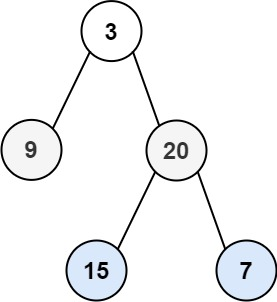# Binary Tree Level Order Traversal

## 1: U-nderstand

Understand what the interviewer is asking for by using test cases and questions about the problem.

• Established a set (2-3) of test cases to verify their own solution later.
• Established a set (1-2) of edge cases to verify their solution handles complexities.
• Have fully understood the problem and have no clarifying questions.
• Have you verified any Time/Space Constraints for this problem?
• Can the input tree be Null?
• Yes, the root node can be Null.
• Do we need to use BFS or DFS for this problem?
• You may use BFS or DFS for this problem. Can you do both?
• What is the space and time complexity?
• Time is `O(N)` and Space is `O(N)`.
``````HAPPY CASE
Input: root = [3,9,20,null,null,15,7]
Output: [,[9,20],[15,7]]````````````Input: root = 
Output: []

EDGE CASE
Input: root = []
Output: []``````

## 2: M-atch

Match what this problem looks like to known categories of problems, e.g. Linked List or Dynamic Programming, and strategies or patterns in those categories.

If you are dealing with Binary Trees some common techniques you can employ to help you solve the problem:

• Think about appropriate Tree Traversal: Pre-Order, In-Order, Post-Order, Level-Order
• Choosing a specific tree traversal helps identify with level-order output
• Store nodes within a HashMap to refer to later
• We don’t have a specific way of referring to previous nodes in a path that could be used in a HashMap. So, a HashMap would not help us as much in this context.
• Using Binary Search to find an element
• We are not working with a Binary Search Tree.
• Applying a level-order traversal with a queue
• Yes, this is the perfect match for this problem.

## 3: P-lan

Plan the solution with appropriate visualizations and pseudocode.

General Idea: Process the binary tree by level using a queue to repeatedly store and process all nodes from previous layer in queue.

``````1. Create a results array to store each level.
2. Create a queue with root node and process until no more nodes in queue
a. Process the current number of nodes in queue to exclude new nodes added to queue.
b. Store value of node into current level and add children from each node to queue, to be processed in next level.
c. Store level into results.
3. Return results.``````

⚠️ Common Mistakes

• Choosing a wrong traversal type

• Try to walk through the problem by hand and see the order in which you are processing the nodes. This will clue you into the type of traversal necessary
• You may use pre-order, post-order, and level order traversal. All you need is to record each level in the correct order. But can you record the in-order traversal in the correct order.
• Failing to recognize the need to store results during the processing of nodes.

## 4: I-mplement

Implement the code to solve the algorithm.

``````# Definition for a binary tree node.
# class TreeNode:
#     def __init__(self, val=0, left=None, right=None):
#         self.val = val
#         self.left = left
#         self.right = right
class Solution:
def levelOrder(self, root: Optional[TreeNode]) -> List[List[int]]:
# Create a results array to store each level.
results = []

# Create a queue with root node and process until no more nodes in queue
queue = [root]
while queue:

# Process the current number of nodes in queue to exclude new nodes added to queue.
levelCount = len(queue)
level = []
for i in range(levelCount):

# Store value of node into current level and add children from each node to queue, to be processed in next level.
node = queue.pop(0)
if node:
level.append(node.val)
queue.append(node.left)
queue.append(node.right)

# Store level into results.
if level:
results.append(level)

# Return results.
return results``````
``````public class Solution {
public List<List<Integer>> levelOrder(TreeNode root) {
// Create a results array to store each level.

if(root == null) return wrapList;

// Create a queue with root node and process until no more nodes in queue
queue.offer(root);
while(!queue.isEmpty()){
// Process the current number of nodes in queue to exclude new nodes added to queue
int levelNum = queue.size();

// Store value of node into current level and add children from each node to queue, to be processed in next level.
for(int i=0; i<levelNum; i++) {
if(queue.peek().left != null) queue.offer(queue.peek().left);
if(queue.peek().right != null) queue.offer(queue.peek().right);
}

// Store level into results
}

// Return results
return wrapList;
}
}``````

## 5: R-eview

Review the code by running specific example(s) and recording values (watchlist) of your code's variables along the way.

• Trace through your code with an input to check for the expected output
• Catch possible edge cases and off-by-one errors

## 6: E-valuate

Evaluate the performance of your algorithm and state any strong/weak or future potential work.

Assume `N` represents the number nodes in tree

• Time Complexity: `O(N)` because we need to visit each node in binary tree.
• Space Complexity: `O(N)` because we need to store each node's val into results.